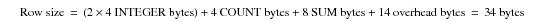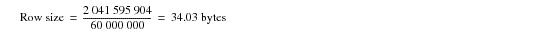# 15.00 - Example Measurement 1 - Teradata Database

prodname
vrm_release
15.00
category
User Guide
featnum
B035-1094-015K

### Example Measurement 1

Table A and Table B both have 60 million 100-byte rows. An aggregate join index with two INTEGER columns and one SUM(INTEGER) column.

From these figures, the computed size of the aggregate join index is as follows:FOR this type of join index … THERE is one join index row … non‑aggregate for every matching row in the base table. aggregate per group of rows in the join of the base tables.

The permanent space specification is as follows:

 Current Permanent Space (Bytes) Peak Permanent Space (Bytes) Maximum Permanent Space (Bytes) 2,041,595,904 2,041,595,904 0

Using these figures, you can compute a measured row size:Because the measured and computed disk spaces are identical within a narrow confidence interval, it is safe to conclude that the disk space formula is accurate for an aggregate join index.